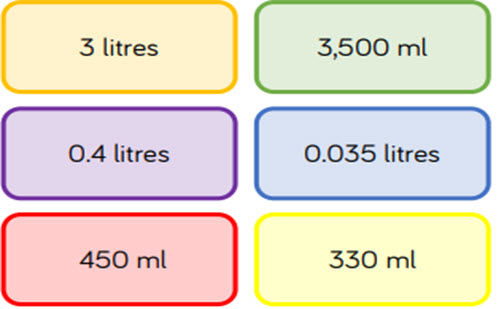# Metric measurement: understanding, converting and calculating

This guide provides a sequence of small steps for exploring measures in context and deepening place value understanding. Focus includes using multiplying and dividing by 10, 100 and 1,000 when converting between units of length, mass and capacity, through to applying understanding and reasoning to solve worded problems.

Year level(s) Year 6
Audience Teacher
Purpose Teaching resource, Teaching strategies
Teaching strategies and pedagogical approaches Questioning, Explicit teaching
Keywords metric units, converting, explicit teaching

## Curriculum alignment

Curriculum connections Numeracy
Strand and focus Measurement, Number, Build understanding
Topics Using units of measurement, Mass and capacity, Place value, Multiplication and division
AC: Mathematics (V9.0) content descriptions
AC9M6M01
Convert between common metric units of length, mass and capacity; choose and use decimal representations of metric measurements relevant to the context of a problem

Numeracy progression Understanding units of measurement (P8)